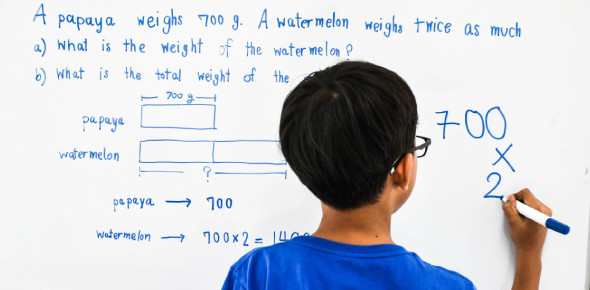# Word Problems Trivia! Ultimate Quiz

10 Questions | Attempts: 214
ShareSettings.

• 1.
7 is approximately what percent of 5?
• A.

58%

• B.

71%

• C.

93%

• D.

133%

• E.

140%

• 2.
Dudley earns \$5.00 per hour as a lifeguard.  He works 25 hours each week and has 15% of his total pay deducted for taxes.  What is his weekly take-home pay?
• A.

\$ 4.25

• B.

\$ 18.75

• C.

\$ 21.25

• D.

\$ 106.25

• E.

\$ 125.00

• 3.
If a/b = 1/7 and b/c = 7/3, then c/a = ?
• A.

1/63

• B.

1/3

• C.

3/21

• D.

21/3

• E.

3

• 4.
Jeff, Susan, and Ben decide to buy a television together.  If the television costs \$500, Jeff pays \$75 and Susan pays 50% of the total price, then Ben pays what fractional part of the cost of the television?
• A.

13/20

• B.

1/2

• C.

2/5

• D.

7/20

• E.

3/20

• 5.
Lauren ran 2 3/8 laps at track practice, while Lizzie ran 3 5/6 laps.  The total distance both ran is within which of the following ranges?
• A.

At least 5 3/4 and less than 6

• B.

At least 6 and less than 6 1/5

• C.

At least 6 1/5 and less than 6 1/4

• D.

At least 6 1/4 and less than 6 1/3

• E.

At least 6 1/3 and less than 6 1/2

• 6.
In a parking lot, there are 3 cars for every 7 SUVs.  If there are 150 vehicles parked in the lot, how many are SUVs?
• A.

10

• B.

15

• C.

45

• D.

105

• E.

150

• 7.
60% of 15% of 800 is what?
• A.

7

• B.

72

• C.

120

• D.

280

• E.

480

• 8.
A pair of boots costs \$50 and a certain coat costs \$150.  If the cost of the boots is increased by 20% and the cost of the coat is decreased by 30%, what is the sum of their new costs?
• A.

165

• B.

200

• C.

225

• D.

265

• E.

330

• 9.
If r = 9, b = 5, and g = -6, what does (r+b-g)(b+g) equal?
• A.

-20

• B.

-8

• C.

8

• D.

19

• E.

20

• 10.
In a group of 48 pianos, 18 are out of tune.  What percentage of the pianos is out of tune?
• A.

18%

• B.

30%

• C.

37 1/2 %

• D.

62 1/2 %

• E.

66 2/3 %

## Related TopicsBack to top
×

Wait!
Here's an interesting quiz for you.